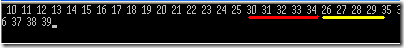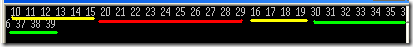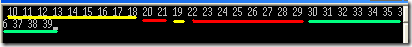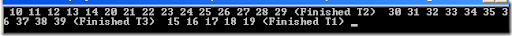# Developing for Multicore machines. Tasks in .NET 4.0 - Why/What/How?

With those multi core processors everywhere, support for parallelism is an already implicit requirement for any new application. This post explores how easily you can implement parallel features in your application, with .NET 4.0.

What is Parallel computing? From Wikipedia,

Parallel computing is a form of computation in which many calculations are carried out simultaneously, operating on the principle that large problems can often be divided into smaller ones, which are then solved concurrently ("in parallel").

Yes, like that photo indicates, parallel systems can do multiple things at the same time.

.NET 4.0 framework provides a wealth of easy to use primitives and abstractions to enable developers to quickly write parallel programs, targeting multi core machines. In this post, we’ll explore Tasks.

The System.Threading.Tasks has all the classes and abstractions you need to develop applications targeting multi core machines.

What is a Task?

A Task in .NET 4.0 is a simple unit of an asynchronous operation. From .NET 4.0 onwards, it is recommended that you should use Tasks instead of creating your own Thread pool work items. Also, it is recommended that you should avoid creating threads, if you don’t need direct control on a thread’s life time.

Using Tasks

You can simply start a task as shown in the below example.

Assume that we want to do some parallel operations, on a range of numbers. For this example, let us keep things simple – So our ProcessNumbers method is not doing anything more than printing the given range of numbers to a console, but I hope you’ll encounter a lot more useful parallel scenarios when you attack a real problem. We are using the Task.Factory.StartNew method to create a task and start it. The first parameter of StartNew method is the action delegate to execute.

You can start a C# Console application in Visual Studio 2010, to compile and run this code instantly.

1. using System;
2. using System.Threading.Tasks;
3.
4. namespace TasksDemo
5. {
6.     class Program
7.     {
8.         static void Main(string[] args)
9.         {
10.             //Create and start first task
11.             var t1=Task.Factory.StartNew(() => ProcessNumbers(10, 20));
12.             //Create and start second task
13.             var t2=Task.Factory.StartNew(() => ProcessNumbers(20, 30));
14.             //Create and start third task
15.             var t3=Task.Factory.StartNew(() => ProcessNumbers(30, 40));
16.             Console.ReadLine();
17.         }
18.
19.         //Some complex processing on the range (we are just printing to console, do something better)
20.         static void ProcessNumbers(int start, int stop)
21.         {
22.             for (int i = start; i < stop; i++)
23.                 Console.Write(" " + i);
24.         }
25.     }
26. }
using System;
using System.Threading.Tasks;

namespace TasksDemo
{
class Program
{
static void Main(string[] args)
{
//Create and start first task
var t1=Task.Factory.StartNew(() => ProcessNumbers(10, 20));
//Create and start second task
var t2=Task.Factory.StartNew(() => ProcessNumbers(20, 30));
//Create and start third task
var t3=Task.Factory.StartNew(() => ProcessNumbers(30, 40));
Console.ReadLine();
}

//Some complex processing on the range (we are just printing to console, do something better)
static void ProcessNumbers(int start, int stop)
{
for (int i = start; i < stop; i++)
Console.Write(" " + i);
}
}
}

And if you run the above program a couple of times and examine the output, you’ll figure it out that the tasks are getting executed asynchronously, in parallel. See the output screen shot, you’ll figure out that our third task (t3) has started printing the results before our second task (t2) has completed. The output may vary in your machine, and with each run.Waiting For Tasks To Finish using Wait()

You can wait for a task to complete, by calling the Wait method of a task. For example, in our above example, if you want to ensure t3 will start only after t2 and t1 are completed, you should modify the code inside our Main(..) method to

1.
2. //Create and start first task
3.  var t1=Task.Factory.StartNew(() => ProcessNumbers(10, 20));
4.  //Create and start second task
5.  var t2=Task.Factory.StartNew(() => ProcessNumbers(20, 30));
6.
7.  //Let us wait till t1 and t2 are completed before starting t3
8.  t1.Wait();
9.  t2.Wait();
10.
11.  //Create and start third task
12.  var t3=Task.Factory.StartNew(() => ProcessNumbers(30, 40));
13.  Console.ReadLine();

//Create and start first task
var t1=Task.Factory.StartNew(() => ProcessNumbers(10, 20));
//Create and start second task
var t2=Task.Factory.StartNew(() => ProcessNumbers(20, 30));

//Let us wait till t1 and t2 are completed before starting t3
t1.Wait();
t2.Wait();

//Create and start third task
var t3=Task.Factory.StartNew(() => ProcessNumbers(30, 40));
Console.ReadLine();

Can you guess what we are doing here? This will ensure that Task t3 will be started only after t1 and t2 are completed. So, if you examine the output, you’ll find that the numbers 30-40 will be printed only after the first two tasks are finished. How ever, you may still see the output of tasks t1 and t2 are intermingled at times, because they are executing in parallel. Here is my output, (t1 – yellow, t2 – red, t3 – green), your output may vary. How ever, with the above code, you have the assurance that task t3 will start only after t1 and t2 are completed, in any machine.

First run.Second runYes, as expected, in both cases you found that Task 3 is executing only after T1 and T2 are finished.

Continuing Tasks

You can request a task to continue with some other operations, once it is done with the current operation. For example, what if you want to notify the user after each task is completed?. Modify the code in our Main(..) method to the following. Please note that in this case, we are no longer waiting for t1 and t2 to complete before starting t3. All t1, t2 and t3 will start in parallel.

1. //Create and start first task
2. var t1=Task.Factory.StartNew(() => ProcessNumbers(10, 20));
3. t1.ContinueWith((t) => { Console.Write(" (Finished T1) "); });
4.
5. //Create and start second task
6. var t2=Task.Factory.StartNew(() => ProcessNumbers(20, 30));
7. t2.ContinueWith((t) => { Console.Write(" (Finished T2) "); });
8.
9. //Create and start third task
10. var t3=Task.Factory.StartNew(() => ProcessNumbers(30, 40));
11. t3.ContinueWith((t) => { Console.Write(" (Finished T3) "); });
12.
13. Console.ReadLine();
            //Create and start first task
var t1=Task.Factory.StartNew(() => ProcessNumbers(10, 20));
t1.ContinueWith((t) => { Console.Write(" (Finished T1) "); });

//Create and start second task
var t2=Task.Factory.StartNew(() => ProcessNumbers(20, 30));
t2.ContinueWith((t) => { Console.Write(" (Finished T2) "); });

//Create and start third task
var t3=Task.Factory.StartNew(() => ProcessNumbers(30, 40));
t3.ContinueWith((t) => { Console.Write(" (Finished T3) "); });

Console.ReadLine();

What will happen? If you run the program, you'll find that the message is printed when a task is finished completely, like this (Your output may vary based on the task completion). At this time, task T2 finished first, then T3 and then T1.Let me run this again. This time, I found that task T1 finished first, then T2 and then T3.

Continuing Tasks based on States of Other Tasks

When you do real life programming, you’ll certainly end up in conditions like “Ok, let us start task ‘X’ when task ‘Y’ and task ‘Z’ are completed”, or “Well, we need to start task ‘P’ when task ‘Q’ or task ‘R’ is completed”.

TaskFactory has a couple of interesting methods that’ll help you in such occasions. Let us go back to our old example. Assume that you want to print 30-40 (i.e, our task 3) only after task 1 and task 2 are completed. Of course, one way is to wait till T1 and T2 is completed (See the example under Waiting For Tasks To Finish using Wait), but here is a better way. Use the ContinueWhenAll method in the TaskFactory, like this.

1. //Create and start first task
2. var t1=Task.Factory.StartNew(() => ProcessNumbers(10, 20));
3.
4. //Create and start second task
5. var t2=Task.Factory.StartNew(() => ProcessNumbers(20, 30));
6.
7. //Start priting 30-40, once t1 AND t2 are finished
8. var t3=Task.Factory.ContinueWhenAll(new Task[] { t1, t2 },
9. (t) => ProcessNumbers(30, 40));
10. Console.Read();
      //Create and start first task
var t1=Task.Factory.StartNew(() => ProcessNumbers(10, 20));

//Create and start second task
var t2=Task.Factory.StartNew(() => ProcessNumbers(20, 30));

//Start priting 30-40, once t1 AND t2 are finished
var t3=Task.Factory.ContinueWhenAll(new Task[] { t1, t2 },
(t) => ProcessNumbers(30, 40));
Console.Read();

The first parameter of ContinueWhenAll(..)  method is an array of tasks, and the second parameter is the action delegate to execute when all tasks in the input array is completed.

Similarly, another interesting method is ContinueWhenAny(..). The following example shows how to start a task, when any of the given set of tasks are finished.

1. //Create and start first task
2.   var t1=Task.Factory.StartNew(() => ProcessNumbers(10, 20));
3.
4.   //Create and start second task
5.   var t2=Task.Factory.StartNew(() => ProcessNumbers(20, 30));
6.
7.   //Start printing 30-40 when t1 OR t2 is finished
8.   var t3=Task.Factory.ContinueWhenAny(new Task[] { t1, t2 },
9.       (t) => ProcessNumbers(30, 40));
10.
11.   Console.Read();
          //Create and start first task
var t1=Task.Factory.StartNew(() => ProcessNumbers(10, 20));

//Create and start second task
var t2=Task.Factory.StartNew(() => ProcessNumbers(20, 30));

//Start printing 30-40 when t1 OR t2 is finished
var t3=Task.Factory.ContinueWhenAny(new Task[] { t1, t2 },
(t) => ProcessNumbers(30, 40));

Console.Read();

You can achieve almost any parallel sequence scenario by nesting ContinueWhenAll(..) and ContinueWhenAny(..)

Returning value from a task

You may use the StartNew<> method to start a task that’ll compute and return a value. For example, assume that you have a function that’ll return the sum of a range, and you want to execute the summation of various ranges in parallel. Here is a quick program that’ll do the same. Have a look at this example.

1. using System;
2. using System.Threading.Tasks;
3.
4. namespace TasksDemo
5. {
6.     class Program
7.     {
8.         static void Main(string[] args)
9.         {
10.             //Create and start first task
11.             var t1 = Task.Factory.StartNew<int>(() => SumRange(10, 20));
12.             //Create and start second task
13.             var t2 = Task.Factory.StartNew<int>(() => SumRange(20, 30));
14.
15.             //Wait till both the tasks finish, sum the results
16.             var allsums = t1.Result + t2.Result;
17.             Console.WriteLine(allsums);
18.             Console.Read();
19.         }
20.
21.         //Sum the numbers
22.         static int SumRange(int start, int stop)
23.         {
24.             int sum = 0;
25.             for (int i = start; i < stop; i++)
26.                 sum += i;
27.             return sum;
28.         }
29.     }
30. }
using System;
using System.Threading.Tasks;

namespace TasksDemo
{
class Program
{
static void Main(string[] args)
{
//Create and start first task
var t1 = Task.Factory.StartNew<int>(() => SumRange(10, 20));
//Create and start second task
var t2 = Task.Factory.StartNew<int>(() => SumRange(20, 30));

//Wait till both the tasks finish, sum the results
var allsums = t1.Result + t2.Result;
Console.WriteLine(allsums);
Console.Read();
}

//Sum the numbers
static int SumRange(int start, int stop)
{
int sum = 0;
for (int i = start; i < stop; i++)
sum += i;
return sum;
}
}
}

Needless to explain, in the above example, we are finding the sum of all values in two value ranges, and then sum the results together. The interesting point to note here is, when you access the computed Result of a task, the task will automatically wait there to complete, so that the computed result can be returned correctly.

I’ll soon write about Parallel Loops and PLINQ, so keep in touch and subscribe to this blog if you are interested. Or, you may follow me in twitter.

Have you read this post about 6 Cool features in Visual Studio 2010 to improve your productivity?

posted @ 2011-09-15 02:49 Net205 Blog 阅读(...) 评论(...)  编辑 收藏